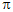# Electrical Engineering - Alternating Current and Voltage

## Why Electrical Engineering Alternating Current and Voltage?

In this section you can learn and practice Electrical Engineering Questions based on "Alternating Current and Voltage" and improve your skills in order to face the interview, competitive examination and various entrance test (CAT, GATE, GRE, MAT, Bank Exam, Railway Exam etc.) with full confidence.

## Where can I get Electrical Engineering Alternating Current and Voltage questions and answers with explanation?

IndiaBIX provides you lots of fully solved Electrical Engineering (Alternating Current and Voltage) questions and answers with Explanation. Solved examples with detailed answer description, explanation are given and it would be easy to understand. All students, freshers can download Electrical Engineering Alternating Current and Voltage quiz questions with answers as PDF files and eBooks.

## Where can I get Electrical Engineering Alternating Current and Voltage Interview Questions and Answers (objective type, multiple choice)?

Here you can find objective type Electrical Engineering Alternating Current and Voltage questions and answers for interview and entrance examination. Multiple choice and true or false type questions are also provided.

## How to solve Electrical Engineering Alternating Current and Voltage problems?

You can easily solve all kind of Electrical Engineering questions based on Alternating Current and Voltage by practicing the objective type exercises given below, also get shortcut methods to solve Electrical Engineering Alternating Current and Voltage problems.

### Exercise :: Alternating Current and Voltage - General Questions

1.

If the rms voltage drop across a 15 kresistor is 16 V, the peak current through the resistor is

 A. 15 mA B. 1.5 mA C. 10 mA D. 1 mA

Answer: Option B

Explanation:

No answer description available for this question. Let us discuss.

2.

The conductive loop on the rotor of a simple two-pole, single-phase generator rotates at a rate of 400 rps. The frequency of the induced output voltage is

 A. 40 Hz B. 100 Hz C. 400 Hz D. indeterminable

Answer: Option C

Explanation:

No answer description available for this question. Let us discuss.

3.

How many degrees are there in/3 rad?

 A. 6° B. 60° C. 180° D. 27°

Answer: Option B

Explanation:

No answer description available for this question. Let us discuss.

4.

To produce an 800 Hz sine wave, a four-pole generator must be operated at

 A. 200 rps B. 400 rps C. 800 rps D. 1,600 rps

Answer: Option B

Explanation:

No answer description available for this question. Let us discuss.

5.

One sine wave has a positive-going zero crossing at 15° and another sine wave has a positive-going zero crossing at 55°. The phase angle between the two waveforms is

 A. 0° B. 45° C. 40° D. none of the above

Answer: Option C

Explanation:

No answer description available for this question. Let us discuss.

#### Current Affairs 2021

Interview Questions and Answers